### It’s Obvious. Not! A Few Answers and More Questions

This installment of “It’s Obvious. Not!” looks at:

Book: “Classical Dynamics of Particles and Systems”

Edition: third

Authors: Jerry B. Marion and Stephen T. Thornton

Publisher: Harcourt Brace Jovanovich

Page: 52

This installation of the series provides a few clarifications into the example presented in the textbook and asks even more questions. I have a feeling that readers steeped in differential equations will immediately follow the reasoning of the example as it is written in the textbook. Please, if you have answers to the remaining questions below, or even ideas, please comment. Thanks!

The first example of the chapter titled “Newtonian Mechanics” asks the reader to find the velocity of an object sliding down a ramp. The solution for the acceleration, (second derivative of the position x), has already been derived as:

The process for deriving the velocity as a function of position illustrated by the author starts with the above equation for acceleration. I’ll simplify by first briefly stating the strategy of the authors solution.

Basic Strategy:

Working from the expression for acceleration, the authors want to demonstrate how to derive velocity as a function of position. They use the following steps that I’ll elaborate on here.

1. Introduce a velocity term to each side of the equation to form a differential equation that can be integrated on each side to solve for velocity in terms of position.
2. Use the product rule of differentiation to re-write the left hand side of the expression so that it only contains velocity.
3. Multiply both sides of the expression by dt so that the integration on the right handside will only integrate over dx producing an x.

We want an equation that relates velocity, (the first derivative of position), to the position of the object on the ramp. Ultimately, the example will manipulate the original expression above so that the left side only contains a differential of velocity and the right side contains a differential of position. When both sides of the expression are integrated, we’ll be left with an expression that relates velocity to position.

Clarifications and Questions:

Now that we know where we’re headed, let’s see how the authors take us there.

The authors multiply both sides of the originalequation by 2 times the velocity, (first derivative of position with respect to time), to give: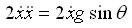and then, in one giant step for physics students everywhere, the authors state on the very next line: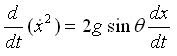Ummm what… !? OK, let’s start at the start. First, why did the author mysteriously decide to multiply both sides of the original equation for acceleration by two times the velocity?

My first thought was why not just integrate the acceleration to get the velocity and be done with it? That would work if we wanted the velocity as a function of time, but we have to solve for it as a function of position. Speaking of time, let’s rewrite the first equation above as:We can do this because acceleration is the derivative of velocity with respect to time. Why we did it is to show explicitly that we have velocity and time so far, but not position. So, we need to somehow introduce position.

Now, returning to the authors original multiplication written in terms of position and velocity:Now, dx is available in the equation. By integrating both sides, we can possibly come up with an answer that provides velocity in terms of position.

This presents the second problem. At present, dx is on both sides of the equation. We’d like to have velocity on one side of the equation and position on the other.

This brings us to the authors’ ‘clever trick’ and another question. The question: Is there a simple mathematical operation to get from

as the book seems to imply by performing this ‘simplification’ in one step? As far as I can tell, there isn’t. Please comment below if you know the way!

That brings us to the authors’ ‘clever trick’. By starting at the end of the simplification and writingasand then using the product rule, (use the first dx/dt as u and the second dx/dt as v), to carry out the derivative with respect to time on the contents of the parenthesis, we get: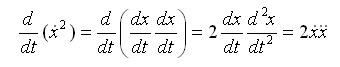So, by ‘cleverly’ choosing to multiply both sides of the original equation by 2 times the velocity, (first derivative of x or position), the authors were left with a quantity on the left-hand-side that is recognizable as the time based derivative of the velocity squared. With that recognition and simplification, the left-hand-side is now written only in terms of velocity.

So, after the ‘clever trick’ that introduced position into the equation and then the ‘clever’ simplification, we’re left at the second step of the example:The authors then multiply both sides by dt and then integrate both sides using the boundary values of the example to arrive at, (the authors’ third step):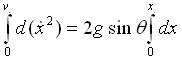This step brings us to the last question. Is there a typo in the third step of the authors’ example? In the text, the upper limit of integration for the left hand side is:

the boundary velocity of the object when it is at position x-naught.

Hopefully, I’ve helped clarify how the authors’ moved from step to step. The following three questions still remain:

1. How did the author decide to multiply both sides of the equation for acceleration by 2 times the velocity?
2. Is there a simple mathematical operation that makes the following equality clear?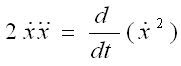3. Is there a typo in the third step of the authors’ example?

Handy StuffAnonymous said…
1) It's a fairly standard thing to do in differential equations...you multiply the whole thing by something that reduces the LHS to a recognisable derivative via product rule.

2. Yes. It's often known as product rule. You wrote it out yourself.

3. No. You missed a point here. The LHS is integrating with respect to velocity squared. When x = x0, v^2 = v0^2, making that the upper limit (and the value of the LHS (+C))

Hope I helped.antigrav_kids said…
This comment has been removed by the author.antigrav_kids said…
Thanks!

Your explanations cleared up everything! Once the reader is aware of the technique you mentioned in your first answer, then it becomes common-place to look for it.

Thanks for the correction on the third question and the confirmation that there isn't a typo.

### Cool Math Tricks: Deriving the Divergence, (Del or Nabla) into New (Cylindrical) Coordinate Systems

Now available as a Kindle ebook for 99 cents! Get a spiffy ebook, and fund more physics
The following is a pretty lengthy procedure, but converting the divergence, (nabla, del) operator between coordinate systems comes up pretty often. While there are tables for converting between common coordinate systems, there seem to be fewer explanations of the procedure for deriving the conversion, so here goes!

What do we actually want?

To convert the Cartesian nabla

to the nabla for another coordinate system, say… cylindrical coordinates.

What we’ll need:

1. The Cartesian Nabla:

2. A set of equations relating the Cartesian coordinates to cylindrical coordinates:

3. A set of equations relating the Cartesian basis vectors to the basis vectors of the new coordinate system:

How to do it:

Use the chain rule for differentiation to convert the derivatives with respect to the Cartesian variables to derivatives with respect to the cylindrical variables.

The chain rule can be used to convert a differe…

### Division: Distributing the Work

Our unschooling math comes in bits and pieces.  The oldest kid here, seven year-old No. 1 loves math problems, so math moves along pretty fast for her.  Here’s how she arrived at the distributive property recently.  Tldr; it came about only because she needed it.
“Give me a math problem!” No. 1 asked Mom-person.

“OK, what’s 18 divided by 2?  But, you’re going to have to do it as you walk.  You and Dad need to head out.”

And so, No. 1 and I found ourselves headed out on our mini-adventure with a new math problem to discuss.

One looked at the ceiling of the library lost in thought as we walked.  She glanced down at her fingers for a moment.  “Is it six?”

“I don’t know, let’s see,” I hedged.  “What’s two times six?  Is it eighteen?”

One looked at me hopefully heading back into her mental math.

I needed to visit the restroom before we left, so I hurried her calculation along.  “What’s two times five?”

I got a grin, and another look indicating she was thinking about that one.

I flashed eac…